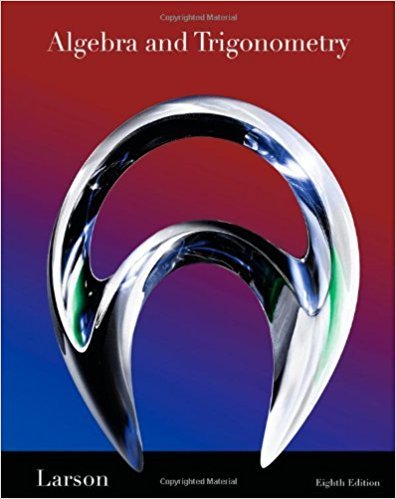×
×

# Solutions for Chapter 4.2: Graphs of Rational Functions## Full solutions for Algebra and Trigonometry | 8th Edition

ISBN: 9781439048474Solutions for Chapter 4.2: Graphs of Rational Functions

Solutions for Chapter 4.2
4 5 0 282 Reviews
27
2
##### ISBN: 9781439048474

Chapter 4.2: Graphs of Rational Functions includes 94 full step-by-step solutions. Since 94 problems in chapter 4.2: Graphs of Rational Functions have been answered, more than 49127 students have viewed full step-by-step solutions from this chapter. This textbook survival guide was created for the textbook: Algebra and Trigonometry, edition: 8. This expansive textbook survival guide covers the following chapters and their solutions. Algebra and Trigonometry was written by and is associated to the ISBN: 9781439048474.

Key Math Terms and definitions covered in this textbook
• Augmented matrix [A b].

Ax = b is solvable when b is in the column space of A; then [A b] has the same rank as A. Elimination on [A b] keeps equations correct.

• Back substitution.

Upper triangular systems are solved in reverse order Xn to Xl.

A sequence of steps (end of Chapter 9) to solve positive definite Ax = b by minimizing !x T Ax - x Tb over growing Krylov subspaces.

• Covariance matrix:E.

When random variables Xi have mean = average value = 0, their covariances "'£ ij are the averages of XiX j. With means Xi, the matrix :E = mean of (x - x) (x - x) T is positive (semi)definite; :E is diagonal if the Xi are independent.

• Determinant IAI = det(A).

Defined by det I = 1, sign reversal for row exchange, and linearity in each row. Then IAI = 0 when A is singular. Also IABI = IAIIBI and

• Distributive Law

A(B + C) = AB + AC. Add then multiply, or mUltiply then add.

• Fast Fourier Transform (FFT).

A factorization of the Fourier matrix Fn into e = log2 n matrices Si times a permutation. Each Si needs only nl2 multiplications, so Fnx and Fn-1c can be computed with ne/2 multiplications. Revolutionary.

• Gauss-Jordan method.

Invert A by row operations on [A I] to reach [I A-I].

• Inverse matrix A-I.

Square matrix with A-I A = I and AA-l = I. No inverse if det A = 0 and rank(A) < n and Ax = 0 for a nonzero vector x. The inverses of AB and AT are B-1 A-I and (A-I)T. Cofactor formula (A-l)ij = Cji! detA.

• Kronecker product (tensor product) A ® B.

Blocks aij B, eigenvalues Ap(A)Aq(B).

• Linear transformation T.

Each vector V in the input space transforms to T (v) in the output space, and linearity requires T(cv + dw) = c T(v) + d T(w). Examples: Matrix multiplication A v, differentiation and integration in function space.

• Network.

A directed graph that has constants Cl, ... , Cm associated with the edges.

• Orthonormal vectors q 1 , ... , q n·

Dot products are q T q j = 0 if i =1= j and q T q i = 1. The matrix Q with these orthonormal columns has Q T Q = I. If m = n then Q T = Q -1 and q 1 ' ... , q n is an orthonormal basis for Rn : every v = L (v T q j )q j •

• Pivot.

The diagonal entry (first nonzero) at the time when a row is used in elimination.

• Polar decomposition A = Q H.

Orthogonal Q times positive (semi)definite H.

• Saddle point of I(x}, ... ,xn ).

A point where the first derivatives of I are zero and the second derivative matrix (a2 II aXi ax j = Hessian matrix) is indefinite.

• Skew-symmetric matrix K.

The transpose is -K, since Kij = -Kji. Eigenvalues are pure imaginary, eigenvectors are orthogonal, eKt is an orthogonal matrix.

• Stiffness matrix

If x gives the movements of the nodes, K x gives the internal forces. K = ATe A where C has spring constants from Hooke's Law and Ax = stretching.

• Symmetric matrix A.

The transpose is AT = A, and aU = a ji. A-I is also symmetric.

• Tridiagonal matrix T: tij = 0 if Ii - j I > 1.

T- 1 has rank 1 above and below diagonal.

×